SYLLABUS  Previous: 4.3.2 Solution of the  Up: 4.3 Methods for European  Next: 4.4 Methods for European

4.3.3 Black-Scholes formula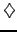[ SLIDE formula - N(x) || same VIDEO as previous section modem - LAN - DSL]

In the case of plain vanilla call and put options, the price can be evaluated in terms of the cumulative normal distribution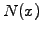and yields the well known Black-Scholes formula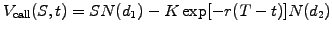(4.3.3#eq.1)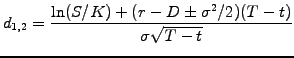(4.3.3#eq.2)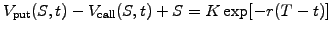(4.3.3#eq.3)

Remember that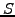denotes the (spot) price of an underlying share that pays a dividend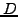and has a historical volatility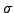,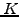is the strike price of the option evolving in time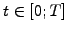from the present to the expiry date and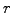the risk-free interest (spot) rate. Note that the last relation (4.3.3#eq.3) is nothing more than the put-call parity previously obtained in (2.1.3#eq.2), where the guaranteed payoff has been discounted back in time to achieve the risk free return of the spot rate. The cumulative normal distribution is related with the so-called error function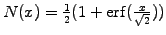, which is available in Matlab and can be approximated with 6 digits accuracy using the polynomial expansion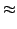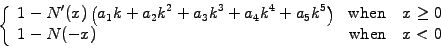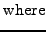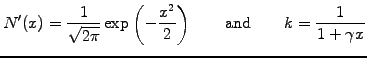(4.3.3#eq.4)

with the coefficients g=0.2316419, a1=0.319381530, a2=-0.356563782, a3=1.781477937, a4=-1.821255978, a5=1.330274429.

SYLLABUS  Previous: 4.3.2 Solution of the  Up: 4.3 Methods for European  Next: 4.4 Methods for European